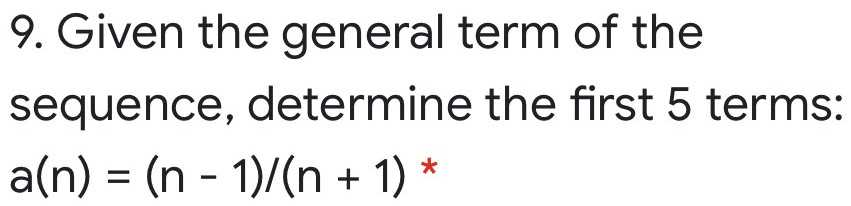### ¿Todavía tienes preguntas de matemáticas?

Pregunte a nuestros tutores expertos
Algebra
PreguntaGiven the general term of the sequence, determine the first $$5$$ terms:

$$a ( n ) = ( n - 1 ) / ( n + 1 )$$ *